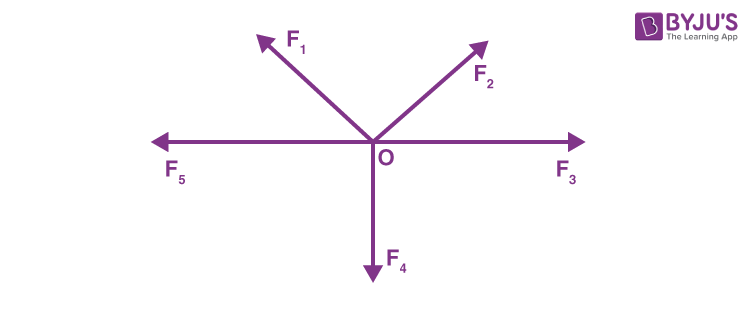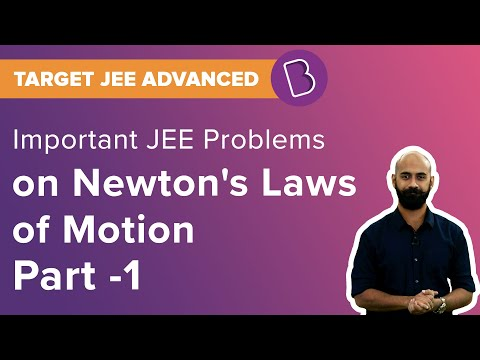# Equilibrium of Concurrent ForcesEquilibrium of a body is a state in which all the forces acting on the body are balanced (cancelled out), and the net force acting on the body is zero. The state of equilibrium is a very important concept to learn in physics. If the net resultant force acting on a body is zero, it means that the net acceleration of the body is also zero (from the second law of motion).

## Types of equilibrium of concurrent forces:

Generally, there are two types of equilibrium:

• 1)  Static equilibrium: This is the type of equilibrium in which the resultant of all the forces acting on the body is zero, i.e. the net acceleration of the body is zero, and the velocity of the body is also zero. It means that the body is at rest. So if a body is at rest and the net acceleration of it is zero, it means the body is in static equilibrium.

Suppose a block is resting on a floor and two forces of 5 N each are acting on it from either side. The forces would cancel each other out and hence the net force on the block would be zero. Since the block is at rest, it will be in static equilibrium.

• 2) Dynamic equilibrium: This is the type of equilibrium in which the resultant of all the forces acting on the body is zero, i.e. the net acceleration of the body is zero, but the velocity of the body is not zero. It means that the body is moving with a constant velocity. So if the net force acting on the body is zero, and it is still moving with some constant velocity, the body is said to be in dynamic equilibrium.

A block attached to a spring under the influence of Simple Harmonic Motion (S.H.M.) is a common example of dynamic equilibrium. At the mean position, the net force acting on the block is zero but the velocity of the block is maximum, which means that the block is in dynamic equilibrium at that point.

Stay tuned with BYJU’S for more such interesting articles. Also, register to “BYJU’S – The Learning App” for loads of interactive, engaging Physics-related videos and an unlimited academic assist.## Frequently Asked Questions – FAQs

Q1

### What is static equilibrium?

This is the type of equilibrium in which the resultant of all the forces acting on the body is zero, i.e. the net acceleration of the body is zero, and the velocity of the body is also zero. It means that the body is at rest. So if a body is at rest and the net acceleration of it is zero, it means the body is in static equilibrium.
Q2

### What is dynamic equilibrium?

This is the type of equilibrium in which the resultant of all the forces acting on the body is zero, i.e. the net acceleration of the body is zero, but the velocity of the body is not zero. It means that the body is moving with a constant velocity. So if the net force acting on the body is zero, and it is still moving with some constant velocity, the body is said to be in dynamic equilibrium.
Q3

### Define equilibrium.

Equilibrium is a state of the body where neither the internal energy nor the motion of the body changes with respect to time.
Q4

### What are the types of force?

Force is a physical cause that can change the state of motion or the dimensions of an object. There are two types of forces based on their applications:
Contact Force
Non-Contact Force
Q5

### Define the line of action of a force.

The line along which a force is acting on an object is called the line of action of the force. The point where the force is acting on an object is called the point of application of the force.
Test your knowledge on Concurrent forces